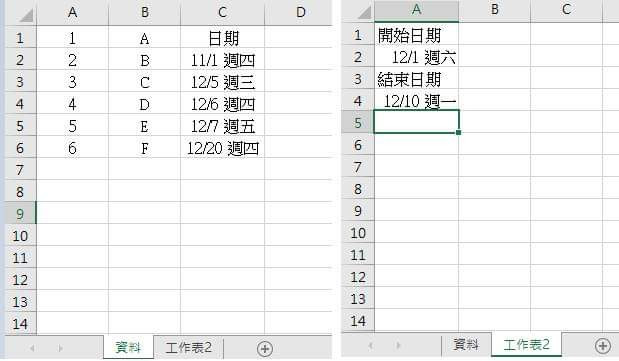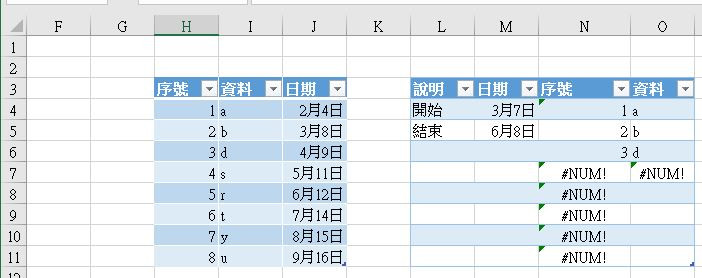#0

## 急～求助 Excel 特定時間抓取資料VBA可以做到,

speoial iT邦新手 5 級 ‧ 2018-12-08 08:35:00 檢舉

### 2 個回答

3`N4=INDEX(\$H\$4:\$H\$11,SMALL(IF((\$J\$4:\$J\$11>=\$M\$4)*(\$J\$4:\$J\$11<=\$M\$5),ROW(\$J\$4:\$J\$11)-ROW(\$J\$4)+1),ROWS(M\$4:M4)))`

`INDEX(資料來源[序號]範圍,SMALL(IF((資料來源[日期]範圍>=開始日)\*(資料來源[日期]範圍<=結束日),ROW(輸出列數)-ROW(輸出資料第一列)+1),ROWS(輸出資料[日期]第一列:該列)))`

'INDEX(資料範圍,列數)取資料第n列
INDEX(資料來源[序號]範圍,
'SMALL(資料範圍,數字)取第N小的值
SMALL(
IF(
(資料來源[日期]範圍>=開始日)*(資料來源[日期]範圍<=結束日),
ROW(資料來源[日期]範圍)-ROW(資料來源[日期]第一列)+1
)
,ROWS(資料來源[日期]第一列:該列)
)
)

``````Sub getrecord()
'宣告
Dim allt, outin As Integer
Dim st, out, std, endd As Range
outin = 0
Set st = Application.Range("H4") '請設定資料來源[日期]第一格
Set out = Application.Range("Q4") '請設定輸出來源左上第一格

Set std = Application.Range("M4") '請設定開始日
Set endd = Application.Range("M5") '請設定結束日
allt = st.End(xlDown).Row()

'清除output
If (out) Then
Range(out, Cells(out.End(xlDown).Row(), out.Column() + 1)).Select
Selection.Clear
End If

'outpput
For ti = st.Row() To allt
comp = Cells(ti, 10).Value
If ((comp >= std) * (comp <= endd)) Then
Debug.Print (Cells(ti, 10))
outin = outin + 1
Cells(ti, 8).Copy Cells(3 + outin, out.Column())
Cells(ti, 9).Copy Cells(3 + outin, out.Column() + 1)

End If
Next ti

End Sub

``````
speoial iT邦新手 5 級 ‧ 2018-12-08 23:55:44 檢舉